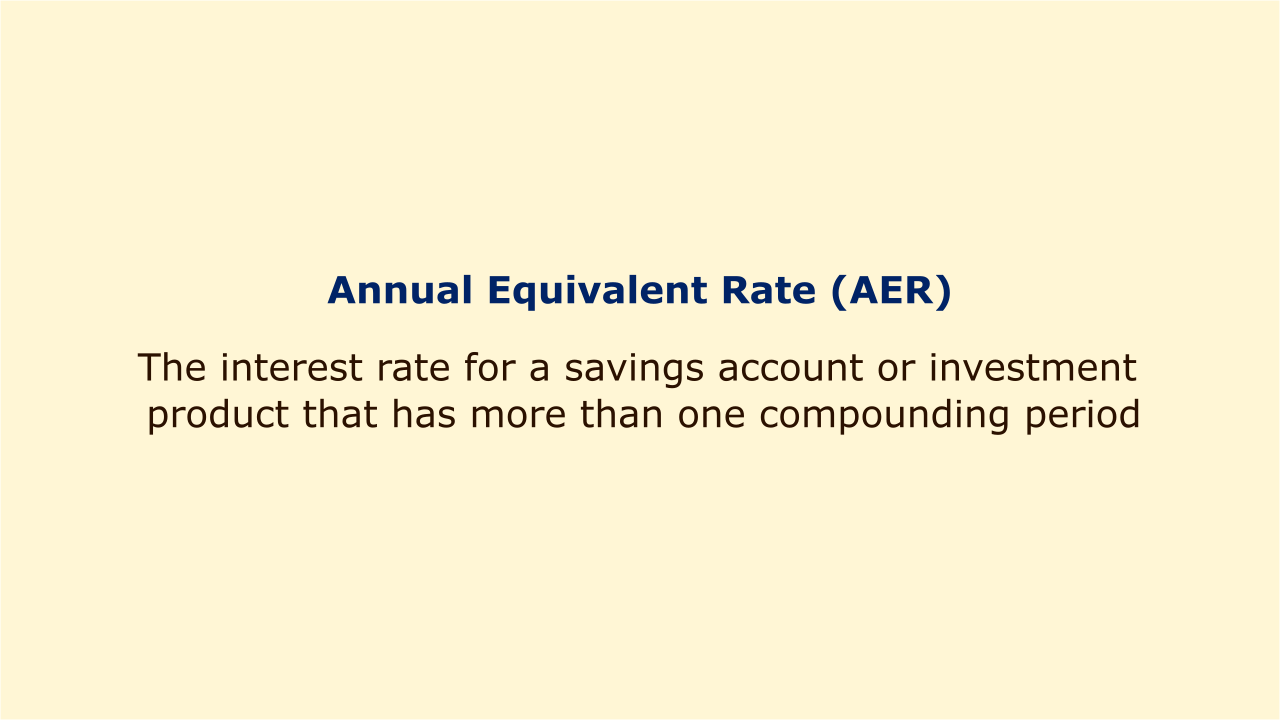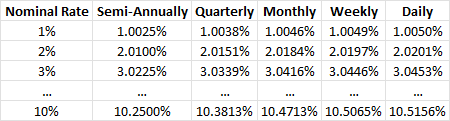# Annual Equivalent Rate (AER)Image: Moneybestpal.com

### The annual equivalent rate (AER) is the interest rate for a savings account or investment product that has more than one compounding period.

The assumption used in calculating AER is that any interest paid will be accounted for in the principal payment's balance and that the subsequent interest payment will be based on the marginally higher account balance. Effective annual interest rate (AER), annual percentage yield (APR), and other terms are also used to refer to AER (APY).

The actual interest rate (AER), which is based on compounding, is what a buyer will actually pay for a loan, investment, or other commodity. The ROI—the actual return of the investment based on compounding, which is higher than the advertised, or nominal, interest rate—is disclosed to investors via the AER.

Assuming that interest is calculated—or compounded—more than once a year, the AER will be higher than the stated interest rate. The difference between the two will increase with the number of compounding periods. Investors can evaluate the AER for various banking products to see whether savings accounts or other investment vehicles offer the best returns.

The formula for the annual equivalent rate is given below:

AER = (1 + r/n)^n - 1

where:
n = The number of compounding periods (times per year interest is paid)
r = The stated interest rate

For example, let's say Bond A offers a semi-annual coupon rate of 3%. The nominal rate of the bond is 6% since it is two 3% coupons. However, the AER of the bond will be higher given that interest is paid out two times a year. Therefore, the AER of the bond will be calculated as:

AER = (1 + 0.06/2)^2 - 1

AER = 6.09%

Bond B, on the other hand, offers a quarterly coupon rate of 1.5%. The nominal rate of the bond is still 6%. However, the AER will be even higher, as the coupons are paid out four times a year.

AER = (1 + 0.06/4)^4 - 1

AER = 6.14%

The table below shows how different compounding frequencies affect the AER for different nominal rates:Image: Moneybestpal.com

The weekly, monthly, half-yearly, or annual compounding periods are used to compare interest rates between loans or investments. The annual equivalent rate is used to compare interest rates between loans or investments. It can therefore be used by both a person looking for the best savings account and an investor comparing bond rates.